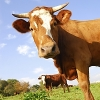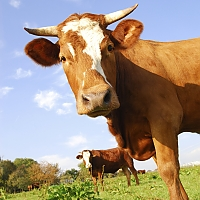# Variables

## Path/URL/Source Variables

The {made} variable returns the relative path (from document root) to the manipulated image (including the filename and extension).

Example:

{exp:ce_img:pair src="/images/example/cow_square.jpg" width="100"}
{/exp:ce_img:pair}

Returns: `/images/made/images/example/cow_square_100_100.jpg`

### orig

The {orig} variable returns the relative path (from document root) to the original image, including the filename. Ex: /images/made/blah.jpg

Example:

{exp:ce_img:pair src="/images/example/cow_square.jpg" width="100"}
{orig}
{/exp:ce_img:pair}

Returns: `/images/example/cow_square.jpg`

The {made_url} variable returns the URL to the manipulated image. Created from your current EE site’s settings. This is essentially a shortcut for path='made'.

Example:

{exp:ce_img:pair src="/images/example/cow_square.jpg" width="100"}
{/exp:ce_img:pair}

Returns: `https://www.causingeffect.com/images/made/images/example/cow_square_100_100.jpg`

### orig_url

The {orig_url} variable returns the URL to the original image. Created from your current EE site’s settings. This is essentially a shortcut for path='orig'. If the original image was a remote image, this will point to the downloaded version of the remote image on your server.

Example:

{exp:ce_img:pair src="/images/example/cow_square.jpg" width="100"}
{orig_url}
{/exp:ce_img:pair}

Returns: `https://www.causingeffect.com/images/example/cow_square.jpg`

### aws_url

If you have the optional CE Image - AWS extension enabled, the `{aws_url}` variable will return the URL to the manipulated image in your bucket. Please see the installation instructions for more details on how to install the extension.

Example:

{exp:ce_img:pair src="/images/example/cow_square.jpg" width="100" bucket="causingeffect"}
{aws_url}
{/exp:ce_img:pair}

Returns: `//causingeffect.s3.amazonaws.com/images/made/images/example/cow_square_100_100.jpg`

### path

The {path} variable will return the full server path of the manipulated image.

Example:

{exp:ce_img:pair src="/images/example/cow_square.jpg" width="100"}
{path}
{/exp:ce_img:pair}

Returns: `/some/server/path/images/made/images/example/cow_square_100_100.jpg`

### path_orig

The {path_orig} variable returns the full server path of the original image. If the original image was a remote image, this will point to the downloaded version of the remote image on your server.

Example:

{exp:ce_img:pair src="/images/example/cow_square.jpg" width="100"}
{path_orig}
{/exp:ce_img:pair}

Returns: `/some/server/path/images/example/cow_square.jpg`

### base64

The {base64} variable will base64 encode the manipulated image so that it can be embedded directly into your HTML.

Example:

{exp:ce_img:pair src="/images/example/cow_square.jpg" width="100"}
<img src="{base64}" width="{width}" height="{height}" alt="" />
{/exp:ce_img:pair}

Returns:### base64_orig

The {base64_orig} variable will base64 encode the original image so that it can be embedded directly into your HTML. Example:

{exp:ce_img:pair src="/images/example/cow_square.jpg" width="100"}
<img src="{base64_orig}" width="{width_orig}" height="{height_orig}" alt="" />
{/exp:ce_img:pair}

Returns:## Dimension Variables

### width

The {width} variable returns the width of the manipulated image.

Example:

{exp:ce_img:pair src="/images/example/cow_square.jpg" width="100"}
{width}
{/exp:ce_img:pair}

Returns: `100`

### width_orig

The {width_orig} variable return the width of the original image.

Example:

{exp:ce_img:pair src="/images/example/cow_square.jpg" width="100"}
{width_orig}
{/exp:ce_img:pair}

Returns: `200`

### height

The {height} variable returns the height of the manipulated image.

Example:

{exp:ce_img:pair src="/images/example/cow_square.jpg" width="100"}
{height}
{/exp:ce_img:pair}

Returns: `100`

### height_orig

The {height_orig} variable returns the height of the original image.

Example:

{exp:ce_img:pair src="/images/example/cow_square.jpg" width="100"}
{height_orig}
{/exp:ce_img:pair}

Returns: `200`

## File Info

### name

The {name} variable returns the name of the manipulated image. For example, if the manipulated image is `cow_100_100.jpg`, then `cow_100_100` will be returned.

Example:

{exp:ce_img:pair src="/images/example/cow_square.jpg" width="100"}
{name}
{/exp:ce_img:pair}

Returns: `cow_square_100_100`

### name_orig

The {name_orig} variable returns the name of the original image. For example, if the original image is `cow.jpg`, then `cow` will be returned.

Example:

{exp:ce_img:pair src="/images/example/cow_square.jpg" width="100"}
{name_orig}
{/exp:ce_img:pair}

Returns: `cow_square`

### extension

The {extension} variable is an extension of the manipulated image. Example: jpg

Example:

{exp:ce_img:pair src="/images/example/cow_square.jpg" width="100"}
{extension}
{/exp:ce_img:pair}

Returns: `jpg`

### extension_orig

The {extension_orig} variable returns the extension of the original image. Example: png

Example:

{exp:ce_img:pair src="/images/example/cow_square.jpg" width="100"}
{extension_orig}
{/exp:ce_img:pair}

Returns: `extension_orig`

### filesize

The {filesize} variable returns the human readable filesize of the manipulated image.

Example:

{exp:ce_img:pair src="/images/example/cow_square.jpg" width="100"}
{filesize}
{/exp:ce_img:pair}

Returns: `13.33 KiB`

### filesize_bytes

The {filesize_bytes} variable returns the filesize of the manipulated image in bytes.

Example:

{exp:ce_img:pair src="/images/example/cow_square.jpg" width="100"}
{filesize_bytes}
{/exp:ce_img:pair}

Returns: `13646`

### filesize_orig

The {filesize_orig} variable returns the human readable filesize of the original image.

Example:

{exp:ce_img:pair src="/images/example/cow_square.jpg" width="100"}
{filesize_orig}
{/exp:ce_img:pair}

Returns: `38.49 KiB`

### filesize_bytes_orig

The filesize of the original image in bytes. `{filesize_bytes_orig}` Example:

{exp:ce_img:pair src="/images/example/cow_square.jpg" width="100"}
{filesize_bytes_orig}
{/exp:ce_img:pair}

Returns: `39414`

### type

The {type} variable returns the image type of the manipulated image. This will always be: `png`, `jpg`, or `gif`.

Example:

{exp:ce_img:pair src="/images/example/cow_square.jpg" width="100" save_type="png"}
{type}
{/exp:ce_img:pair}

Returns: `png`

### type_orig

The {type_orig} variable returns the image type of the original image. This will always be: `png`, `jpg`, or `gif`.

Example:

{exp:ce_img:pair src="/images/example/cow_square.jpg" width="100" save_type="png"}
{type_orig}
{/exp:ce_img:pair}

Returns: `jpg`

## Attribute Variables

Attribute variables are automatically generated from image attributes. They can also be used with the bulk tag.

Example:

{exp:ce_img:bulk crop="yes" width="100" output='<p><img src="{made}" width="{width}" height="{height}" alt="" /><span class="caption">{attr:alt}</span></p>'}
<img src="/images/example/cow_square.jpg" alt="A cow" />
<img src="/images/example/cow-sign.png" alt="A sign" />
{/exp:ce_img:bulk}

Returns:

<p><img src="/images/made/images/example/cow_square_100_100_c1.jpg" width="100" height="100" alt="" /><span class="caption">A cow</span></p>
<p><img src="/images/made/images/example/cow-sign_100_100_c1.png" width="100" height="100" alt="" /><span class="caption">A sign</span></p>

## Content

### ascii_art

The {ascii_art} variable returns the ASCII art text or HTML. This variable is only available in conjunction with the ascii_art parameter.

### average_color

The {average_color} variable returns the hexadecimal color value of the average color of the generated image. The average color will only be calculated if the {average_color} variable is present in the tag data. There is no mechanism in place to cache the result, however, so it is recommended that the native cache="yes" refresh="360" parameters are used (more about performance). See also: top_colors=. Example:

{exp:ce_img:pair src="/images/example/cow_square.jpg" width="100"}
{average_color}
{/exp:ce_img:pair}

Returns: `#a19282`

### top_colors

Used {top_colors} variable is used in conjunction with the top_colors parameter.

## Deprecated Variables

The following variables have been deprecated, but are currently available for use. They may be removed in a future release.

### sized

The {sized} variable returns the relative path (from document root) to the manipulated image. Use made instead.

### w

The {w} variable is an alias for width. This was useful when used with the image gallery module in 1.x, but now that variable prefixing has been introduced, this alias is no longer necessary.

### h

The {h} variable is an alias for height. This was useful when used with the image gallery module in 1.x, but now that variable prefixing has been introduced, this alias is no longer necessary.